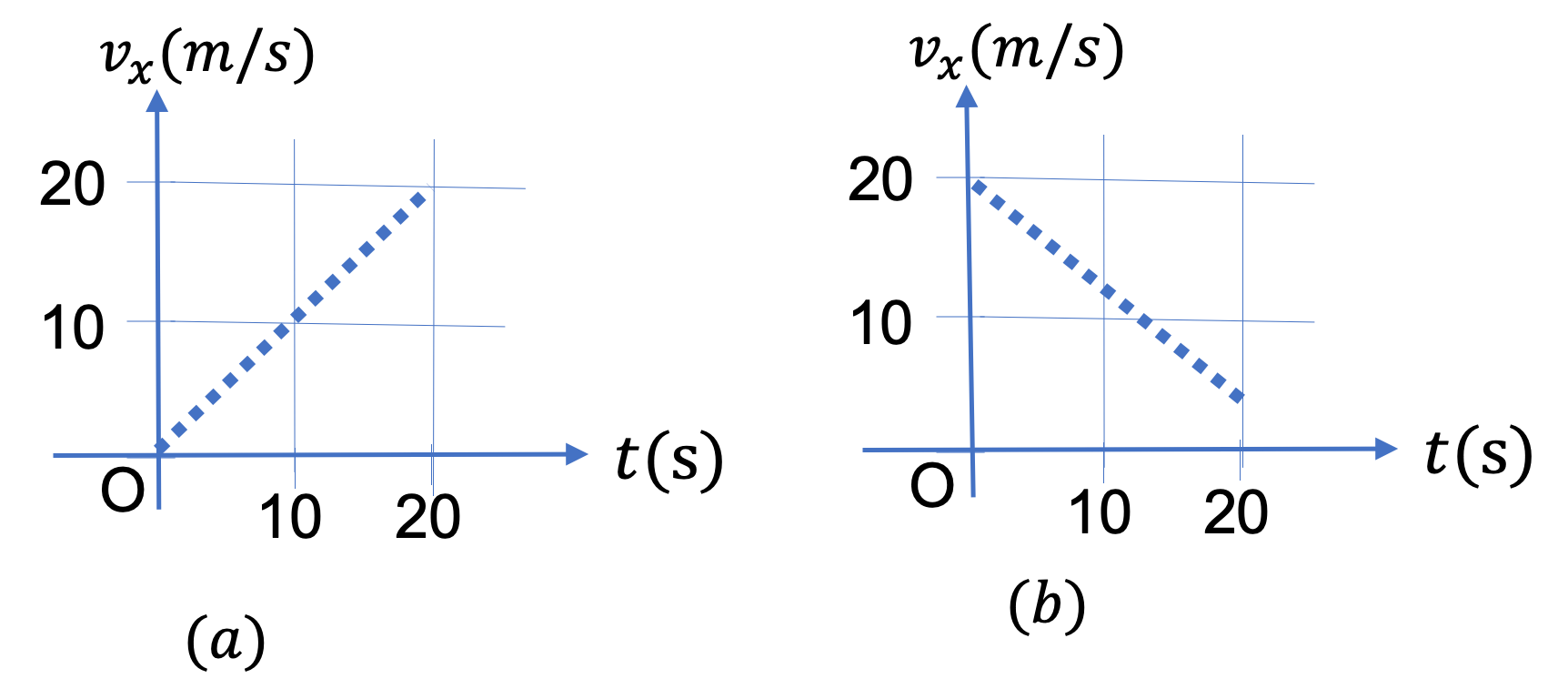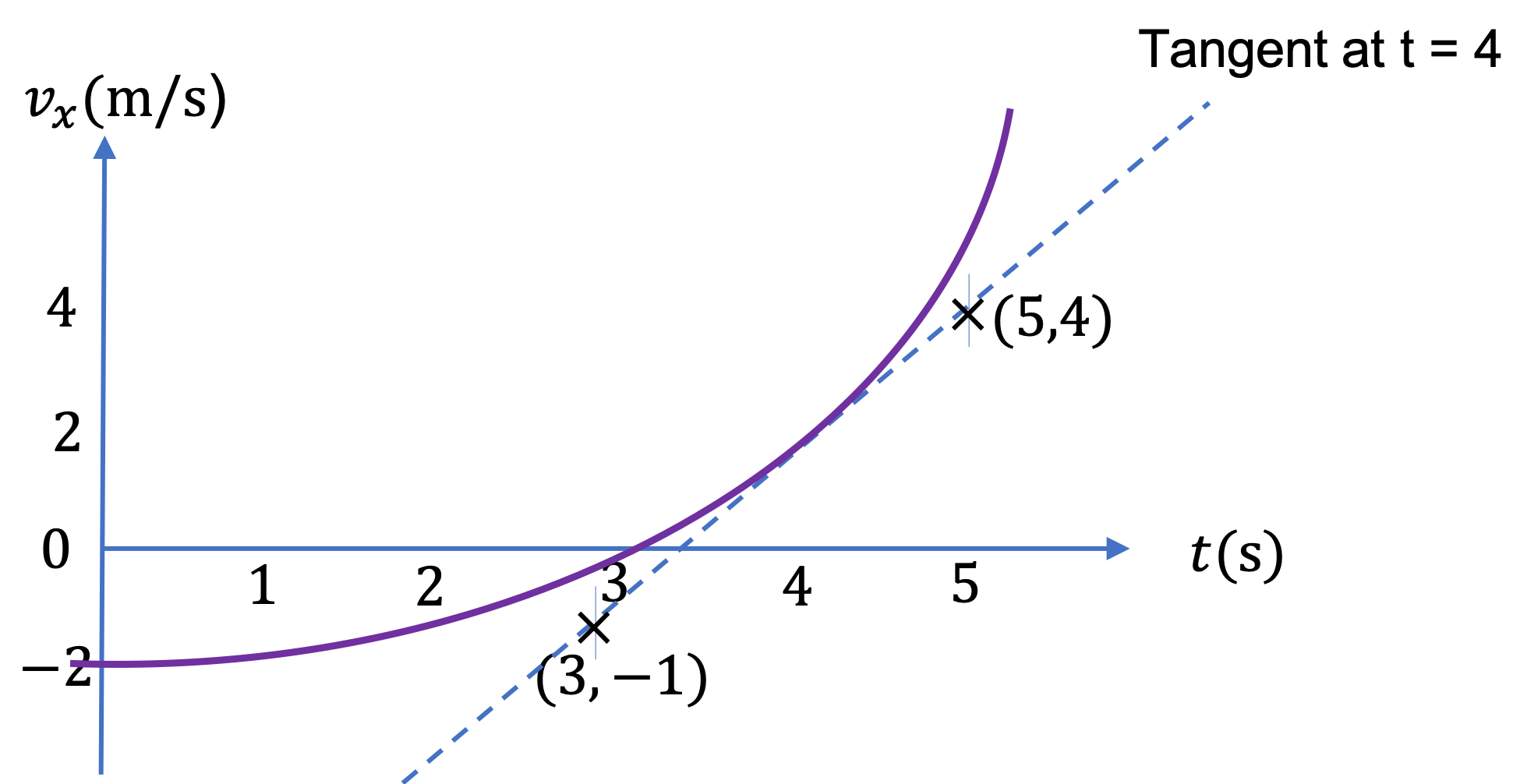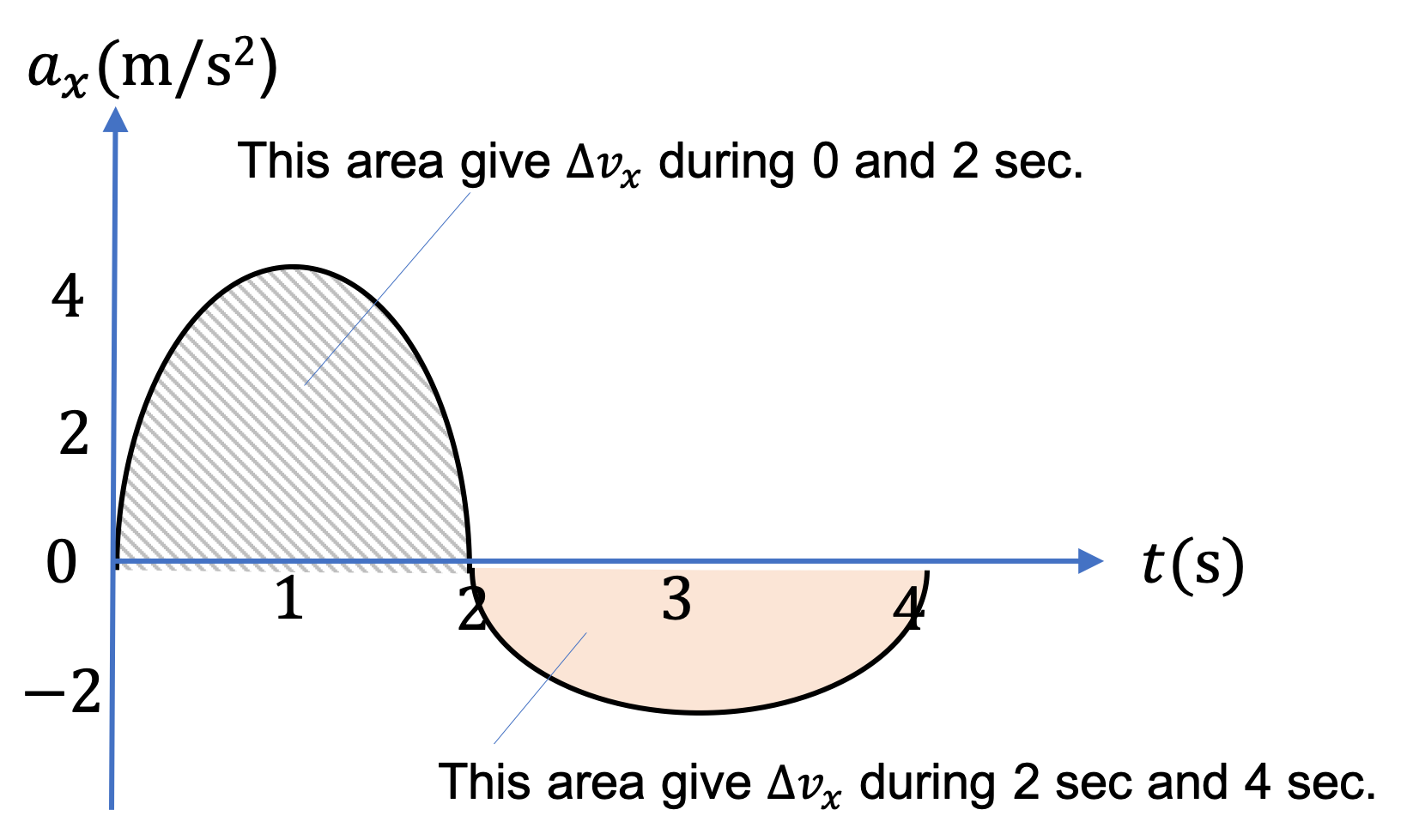## Section2.5Acceleration

The rate at which $x$-coordinate of an object changes with time gave us velocity $v_x\text{.}$ Now, we study the rate at which $v_x$ changes with time. This will give us acceleration for a motion along $x$-axis.

In a later chapter you will learn that the acceleration of an object depends on force you apply on the object. Therefore, it is of considerable interest to understand the concept of acceleration well.

Similar to our treatment of velocity, we will define an average acceleration and an instantaneous acceleration. For a motion on the $x$ axis, we will denote instantaneous acceleration by symbol $a_x$ and average acceleration by $a_{\text{av},x}\text{.}$

### Subsection2.5.1Average Acceleration

The average rate of change of velocity during an interval can be obtained by dividing the change in velocity by the interval. Let $v_{i,x}$ be the velocity at $t_i$ and $v_{f,x}$ be the velocity at $t_f\text{,}$ then average acceleration, $a_{\text{av},x}\text{,}$ will be

\begin{equation} a_{\text{av},x} = \dfrac{v_{f,x} - v_{i,x}}{ t_f - t_i}.\tag{2.5.1} \end{equation}

We often write this as

\begin{equation*} a_{\text{av},x} = \dfrac{\Delta v_x}{ \Delta t}, \end{equation*}

where $\Delta v_x = v_{f,x} - v_{i,x}$ and $\Delta t = t_f - t_i\text{.}$ Note that for average aceleration, we do not need to know what happened at any other instant than just the initial and final instants. For instance, for a motion shown in Figure 2.5.1, the average acceleration between A and B will be obtained from $v_x$ at those points and time between them.

Note that $v_x(\text{at B})$ is negative since the particle is moving towards negative $x$ axis.

\begin{align*} \amp v_x(A) = 2.0\text{ m/s}, \\ \amp v_x(B) = - 2.0\text{ m/s}, \\ \amp a_{\text{av},x} = \frac{-2.0-2.0}{2.0} = -2.0\text{ m/s}^2. \end{align*}

### Subsection2.5.2Instantaneous Acceleration

Often we want acceleration at a particular instant, called instantaneous acceleration, or simply, acceleration. This refers to the average acceleration between that instant, say $t \text{,}$ and an instant very close to $t\text{,}$ e.g., a tiny amount of time later than $t\text{,}$ which we can indicate by $t + \Delta t \text{.}$ Or, alternately, between $t$ and a tiny instant before $t\text{,}$ say at $t-\Delta t\text{.}$

How close instants $t$ and $t + \Delta t$ be? The answer is as close as possible except right at $t\text{.}$ This is the notion of infintesimal interval already discussed in Subsection 2.4.3 on instantaneous velocity.

Denoting instantaneous acceleration for a motion along $x$ axis by symbol $a_x \text{,}$ we write this special averaging formally using the notion of a limit in Calculus,

\begin{equation} a_x = \lim_{\Delta t \rightarrow 0}\dfrac{v_x\left(\text{at } t+\Delta t\right) - v_x\left(\text{at } t\right)}{\Delta t},\label{eq-instantaneous-acceleration-1d-limit}\tag{2.5.2} \end{equation}

which is also denoted by the derivative symbol $dv_x/dt\text{.}$

\begin{equation} a_x = \dfrac{dv_x}{dt}.\tag{2.5.3} \end{equation}

Graphically, this derivative can be computed by the slope of the tangent to the $v_x$ versus $t$ plot at the instant of interest.

Positive and Negative $a_x$ When Moving Towards $x = + \infty\text{:}$ When an object is moving towards $x = + \infty$ with increasing speed, we will have $v_x\left(\text{at } t+\Delta t\right) \gt v_x\left(\text{at } t\right)\text{,}$ which will give positive $a_x\text{.}$ And, when the object is moving towards $x = + \infty$ with decreasing speed, we will have $v_x\left(\text{at } t+\Delta t\right) \lt v_x\left(\text{at } t\right)\text{,}$ which will give negative $a_x\text{.}$

Positive and Negative $a_x$ When Moving Towards $x = - \infty\text{:}$ When the object is moving towards $x = - \infty\text{,}$ $v_x \lt 0\text{.}$ That means that if the motion is with increasing speed, we will have $v_x\left(\text{at } t+\Delta t\right) \lt v_x\left(\text{at } t\right)\text{,}$ which will give negative $a_x\text{.}$ And, when the object is moving towards $x = - \infty$ but with decreasing speed, we will have $v_x\left(\text{at } t+\Delta t\right) \gt v_x\left(\text{at } t\right)\text{,}$ which will give positive $a_x\text{.}$

### Subsection2.5.3Graphical Definition of Acceleration

Since the slope of a function is the rate of change of that function, the rate of change of velocity at a particular instant can be obtained from the slope of the tangent of the velocity versus time plot. We can take this as defining acceleration $a_x$ for one-dimensional motion on the $x$ axis.

\begin{equation} a_x = \text{ slope of tangent to }v_x\text{ versus }t.\tag{2.5.4} \end{equation}

If the plot of $v_x$ versus $t$ is linear, i.e., a straight line, then the tangent line is same as the line of the plot, hence $a_x$ is just the slope of the line.Figure 2.5.2. The slope of $v_x$ versus $t$ plot gives the acceleration $a_x \text{.}$ Since the plot is linear, the slope is same for all instants plotted. In (a) $a_x = 1.0 \text{ m/s}^2$ and in (b) $a_x = - 0.75 \text{ m/s}^2\text{.}$

But, if the plot of $v_x$ versus $t$ is curved, then you would draw a tangent line at the instant of interest, and compute the slope of the tangent line to get $a_x \text{.}$Figure 2.5.3. The slope of $v_x$ versus $t$ plot gives the acceleration $a_x \text{.}$ Since the plot is not linear, we need to first draw a tangent line at the instant we want the acceleration value. The figure illustrates $a_x$ at $t = 4\text{ sec}\text{,}$ where the slope of the tangent line is $a_x = (4-(-1))/(5-3) = 2.5\text{ m/s}^2 \text{.}$

From the $v_x$ versus $t$ plot in Figure 2.5.5, find $a_x$ at the following instants: (a) $t = 1\text{ sec}\text{,}$ (b) $t = 3\text{ sec} \text{,}$ and (c) $t = 3.75\text{ sec} \text{.}$

Hint

Use slopes.

(a) $0\text{,}$ (b) $-4\text{ m/s}^2\text{,}$ (c) $4\text{ m/s}^2$

Solution

(a) The $v_x$ versus $t$ plot through the time when $t = 1\text{ sec}$ is a straight line. Therefore, $a_x$ will simply be slope of this part of the plot. The slope of this line is clearly zero. Therefore, $a_x (\text{at }t=1\text{ sec}) = 0\text{.}$

(b) The $v_x$ versus $t$ plot through the time when $t = 3\text{ sec}$ is a straight line. Therefore, $a_x$ will simply be slope of this part of the plot. The slope of this line is obtained by picking two points on the line between $t = 2\text{ sec}$ and $t = 3.5\text{ sec}\text{.}$ We pick points at $t = 2\text{ sec}$ and $t = 3\text{ sec}$ to obtain

\begin{equation*} a_x = \dfrac{0 - 4\text{ m/s}}{3\text{ s}-2\text{ s}} = -4\text{ m/s}^2. \end{equation*}

(b) The $v_x$ versus $t$ plot through the time when $t = 3.75\text{ sec}$ is a straight line. Therefore, $a_x$ will simply be slope of this part of the plot. The slope of this line is obtained by picking two points on the line between $t = 3.5\text{ sec}$ and $t = 4.0\text{ sec}\text{.}$ We pick points at $t = 3.5\text{ sec}$ and $t = 4\text{ sec}$ to obtain

\begin{equation*} a_x = \dfrac{0 - (-2)\text{ m/s}}{4\text{ s}-3.5\text{ s}} = 4\text{ m/s}^2. \end{equation*}

### Subsection2.5.4$\Delta v_x$ from $a_x$ Graphically

Since $a_x$ is detivative of $v_x(t)\text{,}$ a change in $v_x$ will be given by an integral of $a_x(t)\text{.}$ Since we can think of an integral as area under a plot of $a_x$ between the $a_x(t)$ and $a_x=0$ as illustrated in Figure 2.5.6.. We have seen same relation between $v_x$ and $x(t)$ as we are seeing between $a_x$ and $v_x(t)\text{.}$

The area under the curve is the area between the graph and $a_x=0$ line. Therefore, from positive $a_x$ we get positive area and for negative $a_x$ we get negative area. Positive $a_x$ leads to positive change in the $v_x$ and a negative $a_x$ to a negative change in $v_x\text{.}$ This makes sense; say, starting $v_x = 10\text{ m/s}$ and acceleration is constant, $a_x=-3\text{ m/s}^2\text{.}$ Then, change in velocity in $2\text{ sec}$ will be $\Delta v_x = -3\times 2 = -6\text{ m/s}\text{.}$ This will give $v_x = 4\text{ m/s}$ after $2\text{ sec}\text{.}$Figure 2.5.6. The area under the curve to get $\Delta v_x$ from $a_x$ versus $t$ plot. The area for the curve when $a_x \gt 0$ is positive, as between $t=0$ and $t= 2\text{ sec}$ and the area for the curve when $a_x \lt 0$ is negative, as between $t= 2\text{ sec}$ and $t= 4\text{ sec}\text{.}$

A ball is initially ($t=0$) moving at $v_x = 3\text{ m/s}\text{.}$ The acceleration of the ball at different times are as shown in the $a_x$ versus $t$ plot in Figure 2.5.8. Find $v_x$ at (a) $t = 1\text{ sec} \text{,}$ (b) $t = 2\text{ sec} \text{,}$ and (c) $t = 4\text{ sec} \text{.}$

Hint

Use area-under-curve.

(a) $5\text{ m/s}\text{,}$ (b) $5\text{ m/s}$ , (c) $1\text{ m/s} \text{.}$

Solution

(a) From the area-under-curve we can find the change in $v_x$ during $t= 0$ to $t = 1\text{ sec}\text{.}$ Adding this change to the $v_x$ at $t= 0$ will give us the $v_x$ at $t=1\text{ sec}\text{.}$

\begin{equation*} \Delta v_x = \dfrac{1}{2}\times 4 \times 1 = 2\text{ m/s}. \end{equation*}

Therefore, $v_x$ at $t=1\text{ sec}\text{:}$

\begin{equation*} v_x(\text{ at }t=1\text{ sec }) = v_x(\text{ at }t=0\ ) + \Delta v_x = 5\text{ m/s}. \end{equation*}

(b) The area-under-the-curve during $t= 1\text{ sec}$ to $t = 2\text{ sec}$ is zero. Hence the velocity does not change over this interval, i.e., $\Delta v_x = 0 \text{.}$

\begin{equation*} v_x(\text{ at }t=2\text{ sec }) = v_x(\text{ at }t=1\text{ sec } ) + \Delta v_x = 5\text{ m/s}. \end{equation*}

(c) From the area-under-curve we can find the change in $t= 2\text{ sec}$ to $t = 4\text{ sec}\text{.}$ Adding this change to the $v_x$ at $t= 2\text{ sec}$ will give us the $v_x$ at $t=4\text{ sec}\text{.}$

\begin{equation*} \Delta v_x = -2 \times (4-2) = -4\text{ m/s}. \end{equation*}

Therefore, $v_x$ at $t=4\text{ sec}\text{:}$

\begin{equation*} v_x(\text{ at }t=4\text{ sec }) = v_x(\text{ at }t=2\text{ sec } ) + \Delta v_x = 1\text{ m/s}. \end{equation*}

### Subsection2.5.5(Calculus) Analytic Definition of Acceleration

The derivative of velocity with respect to time gives the rate at which velocity changes. That is, acceleration $a_x$ on the $x$ axis is the derivative of the velocity $v_x \text{.}$

\begin{equation} a_x = \dfrac{dv_x}{dt}.\label{eq-1d-review-accleration-def}\tag{2.5.5} \end{equation}

In theoretical work you might find that you are given position as a function $x(t) \text{.}$ For example, a block attached to a spring oscillates with its position $x(t) = (5 \text{ cm}) \cos( 2\pi t)\text{,}$ where $t$ is in seconds. If you plot this $x$ versus $t \text{,}$ you will notice that the block moves between $x = - 5$ cm and $x = 5$ cm, repeating a complete cycle every second.

(a) What is the velocity of this block at (i) $t = 0.25$ s, (ii) $t = 0.5$ s, and (iii) $t = 0.75$ s.

(b) What is the acceleration of this block at (a) $t = 0.25$ s, (b) $t = 0.5$ s, and (c) $t = 0.75$ s.

Hint

Take the derivatives: (a) $v_x = dx/dt\text{,}$ (b) $a_x = dv_x/dt\text{.}$

(a) (i) $-10\pi\text{ cm/s}\text{,}$ (ii) $0\text{,}$ (iii) $10\pi\text{ cm/s}\text{,}$ (b) (i) $0\text{,}$ (ii) $20\pi^2 \text{ cm/s}^2\text{,}$ (iii) $0\text{.}$

Solution 1 (a)

(a) Taking the derivative of given $x(t)$ and then evaluating the result at the required times should give us the answers.

\begin{equation*} v_x = \dfrac{dx}{dt} = (-10\pi \text{ cm/s}) \sin(2\pi t). \end{equation*}

(i) $v_x = (-10\pi \text{ cm/s}) \sin(2\pi \times 0.25) = -10\pi \text{ cm/s} = -31.4 \text{ cm/s}.$

(ii) $v_x = (-10\pi \text{ cm/s}) \sin(2\pi \times 0.50) = 0.$

(iii) $v_x = (-10\pi \text{ cm/s}) \sin(2\pi \times 0.75) = 10\pi \text{ cm/s}= 31.4 \text{ cm/s}.$

Solution 2 (b)

(b) Taking the derivative of $v_x$ and then evaluating the result at the requires times should give us the answers.

\begin{equation*} a_x = \dfrac{dv_x}{dt} = (-20\pi^2 \text{ cm/s}^2) \cos(2\pi t). \end{equation*}

(i) $a_x = (-20\pi^2 \text{ cm/s}^2) \cos(2\pi \times 0.25) = 0.$

(ii) $a_x = (-20\pi^2 \text{ cm/s}^2) \cos(2\pi \times 0.50) = 20\pi^2 \text{ cm/s}^2.$

(iii) $a_x = (-20\pi^2 \text{ cm/s}^2) \cos(2\pi \times 0.75) = 0.$

### Subsection2.5.6(Calculus) Change in Velocity from Acceleration Analytically

The inverse problem of obtaining change in velocity, $\Delta v_x\text{,}$ from a known acceleration, $a_x(t) \text{,}$ is a similar problem of going from $v_x$ to $\Delta x\text{.}$ Therefore, the math is similar.

The inverse of Eq. (2.5.5) gives the change in velocity $\Delta v_x = v_{f,x} - v_{i,x}$ when we integrate acceleration $a_x \text{.}$

\begin{equation} \Delta v_x \equiv v_{f,x} - v_{i,x} = \int_{t_i}^{t_f}\ a_x(t)\, dt.\label{eq-dvx-as-integral-of-ax}\tag{2.5.6} \end{equation}

In this equation, if we choose $t_f$ to be a variable $t\text{,}$ then we will get velocity as a function of $t\text{.}$ Writing $v_{f,x}$ as $v_x(t)$ we have

\begin{equation} v_{x}(t) = v_{i,x} + \int_{t_i}^{t}\ a_x(t')\, dt',\label{eq-vx-as-integral-of-ax}\tag{2.5.7} \end{equation}

where $v_{i,x}$ is some known velocity at the initial instant and I have replaced the integration variable by symbol $t'$ so as not to get confused by $t\text{.}$ Integrating $v_x$ as a function we will get the change in position.

\begin{align*} x_f - x_i &= \int_{t_i}^{t_f} v_x(t) dt \\ &= \int_{t_i}^{t_f} \left[ v_{i,x} + \int_{t_i}^{t}\ a_x(t')\, dt' \right] dt \\ &= v_{i,x}\left(t_f - t_i \right) + \int_{t_i}^{t_f} \left( \int_{t_i}^{t}\ a_x(t')\, dt' \right) dt \end{align*}

Let us work out the case of a constant acceleration. Let us say that acceleration has a constant value $a_x=a_0\text{,}$ initial instant $t_i=0\text{,}$ and $v_{i,x}=v_0\text{.}$ Let us write $t_f-t_i$ as $T\text{.}$ Then, we will get the following for change in $x\text{.}$

\begin{align*} x_f - x_i &= v_{0} T + \int_{0}^{T} \left( a_0\, t \right) dt \\ &= v_{0} T + \frac{1}{2} a_0 T^2 \end{align*}

The position of a block on a spring along $x$ axis is given by

\begin{equation*} x(t) = A\, cos(\omega\, t + \phi), \end{equation*}

where $A\text{,}$ $\omega\text{,}$ and $\phi$ are constant. Show that its acceleration at an arbitrary instant $t$ is given by

\begin{equation*} a_x = -\omega^2\, x(t). \end{equation*}
Hint

Differentiate $x\text{.}$

Solution

Not provided.

The acceleration of a car at the start is changing with time, as given by the following function.

\begin{equation*} a(t) = 12.0\; t^2. \end{equation*}

The unit of acceleration is $\text{m/s}^2\text{.}$ If the car starts from rest, how far will it go in $3.0\text{ sec}\text{?}$ Place the motion of the car along $x$ axis.

Hint

Integrate $a_x\text{,}$ then integrate again.

$81.0\text{ m}\text{.}$